# An Introduction to Differential Proportionality

## Introduction

In this vignette, we use a real dataset to show how we can apply differential proportionality analysis to understand RNA-seq count data. We place a particular emphasis here on documenting the differential proportionality measures available through this package. This vignette discusses the propd method.

## Theory

Let us consider two non-zero positive vectors, $$X$$ and $$Y$$, measuring the relative abundance of raw sequence counts. One way to understand whether two vectors associate with one another is to consider the variance of the log-ratios (VLR), a compositionally valid measure of association that makes up part of the definition of the proportionality metrics. Although we can calculate VLR using log-ratio transformed data, we see that in this case the denominator factor (e.g., the geometric mean or unchanged reference) cancels. As such, we can use these counts without any transformation:

$\textrm{VLR(X, Y)} = \textrm{var}(\log(\textrm{X} - \textrm{Y}))$

The propd method uses the VLR to test for differential proportionality. Recall that the reason we do not use the VLR for proportionality analysis is that we cannot compare the VLR for one pair against the VLR for another pair (thus why we defined $$\phi$$ and $$\rho$$ as a modification to the VLR that gives it scale). However, in differential proportionality analysis, we compare the VLR for one pair across groups. Specifically, we extract the fraction of variance (interpreted as the residual variance of proportional features) where proportionality holds in both or only one of two groups.

We consider here two forms of differential proportionality. The first, which we call disjointed proportionality, considers the case where the proportionality of a pair holds in both groups, but the ratio between the partners changes between the groups (i.e., the slope of the proportionality changes). The second, which we call emergent proportionality, considers the case where there is proportionality in only one of the groups (i.e., the strength of the proportionality changes).

Given two groups, sized $$k$$ and $$n - k$$, we define $$\theta_d$$, a measure of disjointed proportionality, as the pooled (weighted) VLR within the two groups divided by the total VLR:

$\theta_d(\textrm{X}, \textrm{Y}) = \frac{(k-1)\textrm{VLR}_1 + (n-k-1)\textrm{VLR}_2}{(n-1)\textrm{VLR}}$

Likewise, we define $$\theta_e$$, a measure of emergent proportionality, as the fraction of variance that remains when subtracting the fraction of the dominating group variance:

$\theta_e(\textrm{X}, \textrm{Y}) = 1 - \frac{\mathrm{max}[(k-1)\textrm{VLR}_1,(n-k-1)\textrm{VLR}_2]}{(n-1)\textrm{VLR}}$

## Handling of zero values

Note that as a consequence of using the logarithm, we cannot compute VLR in the setting of zero counts. How to best handle zeros in compositional data analysis remains an area of active research. Analysts should carefully evaluate the effect that this has on their results.

By default, propd takes the simplest approach of replacing all zero values with 1. Alternatively, the propd method offers the option to use a transformation to approximate VLR based on a parameter $$\alpha$$ that is usually close to zero. We define aVLR as an approximation of VLR, used in place of VLR in any $$\theta$$ equation:

$\textrm{aVLR(X, Y)} = \frac{\sum\left(\frac{\textrm{X}^{\alpha}}{\textrm{mean}(\textrm{X}^{\alpha})} - \frac{\textrm{Y}^{\alpha}}{\textrm{mean}(\textrm{Y}^{\alpha})}\right)^2}{(n-1)\alpha^2}$

## False discovery rate

The propd method does not return a vector of p-values like most statistical tests. Instead, the user may choose to estimate the false discovery rate (FDR) using permutations of the group assignments to generate an empiric distribution of $$\theta$$ values (specific to the supplied data). With this, for each arbitrary cutoff of $$\theta$$, the FDR is calculated as the average random number of pairs with $$\theta<\textrm{cutoff}$$ divided by the observed number of pairs with $$\theta<\textrm{cutoff}$$. This uses the updateCutoffs method, like propr.

Alternatively, it is possible to calculate an F-statistic, as discussed in the next vignette:

$F = (n-2)\frac{1-\theta_d}{\theta_d}$

## Implementation

The propd function estimates differential proportionality by calculating $$\theta$$ for all feature pairs, with or without the aid of the aVLR-transformation. This function takes the following arguments as input and returns a propd object as a result:

• counts: a matrix of $$n$$ samples (as rows) and $$d$$ features (as columns)
• group: an $$n$$-dimensional vector corresponding to subject labels
• alpha: an optional argument to trigger and guide transformation
• p: the total number of permutations used to estimate FDR

Below, we run propd using the iris dataset.

library(propr)
data(iris)
keep <- iris\$Species %in% c("setosa", "versicolor")
counts <- iris[keep, 1:4] * 10
group <- ifelse(iris[keep, "Species"] == "setosa", "A", "B")
pd <- propd(counts, group, alpha = NA, p = 100)

The resultant propd object contains both $$\theta_d$$ and $$\theta_e$$ metrics (among others), although only $$\theta_d$$ is active by default. While a $$\theta$$ is active, it forms the basis for permutation testing (i.e., FDR estimation) and visualization. Users can easily change which $$\theta$$ is active using the functions setDisjointed and setEmergent.

theta_d <- setDisjointed(pd)
theta_e <- setEmergent(pd)

Once the $$\theta$$ of interest is active, the user can estimate FDR using the updateCutoffs function.

theta_d <- updateCutoffs(theta_d, cutoff = seq(0.05, 0.95, 0.3))
## |------------(25%)----------(50%)----------(75%)----------|
theta_e <- updateCutoffs(theta_e, cutoff = seq(0.05, 0.95, 0.3))
## |------------(25%)----------(50%)----------(75%)----------|

In order to reduce RAM overhead, the propd object never stores the intermediate $$\theta$$ values for permutation testing. However, when a propd object is created, it contains all the randomized group assignments needed for permutation testing, meaning that each updateCutoffs run effectively uses the same random seed. One could exploit the data contained in the @permutes slot to reproduce the intermediate calculations if needed.

## Sample data

To understand differential proportionality, we use two propd objects, called pd.d (for $$\theta_d$$) and pd.e (for $$\theta_e$$), built from the bundled caneToad.counts RNA-seq data (Rollins 2015). Specifically, we use the results of the propd function as applied to cane toad transcripts with at least 40 counts in all 20 samples (thus removing any transcripts with 0 counts), subsetted to include only pairs with the top 1000 smallest $$\theta$$.

Note that in this vignette, we never apply updateCutoffs to either data object. When estimating FDR, it is necessary to use an unfiltered propd object to keep estimations unbiased.

data(pd.d, package = "propr") # top 1000 disjointed pairs
data(pd.e, package = "propr") # top 1000 emergent pairs

## Disjointed proportionality

We begin now by looking at disjointed proportionality in more detail. Based on its definition, we see that low values of $$\theta_d$$ select pairs where the total VLR far exceeds the weighted sum of the within-group VLRs. Often, the within-group VLRs are about the same size. However, this is not a requirement so long as the within-group VLRs are both small compared to the total VLR.

Below, we use the getResults function to tabulate important pairwise measurements. Then, we show a scatter plot of the abundance for features “39” and “37”, as colored by the experimental group, with the slopes of the trend lines equal to the ratio means.

tab <- getResults(pd.d)
plot(pd.d@counts[, 39], pd.d@counts[, 37], col = ifelse(pd.d@group == "WA", "red", "blue"))
grp1 <- pd.d@group == "WA"
grp2 <- pd.d@group != "WA"
abline(a = 0, b = pd.d@counts[grp1, 37] / pd.d@counts[grp1, 39], col = "red")
abline(a = 0, b = pd.d@counts[grp2, 37] / pd.d@counts[grp2, 39], col = "blue")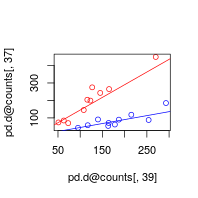Here, we see that these two features change proportionally across the samples within each group (as expected based on their small values of $$\textrm{VLR}_1$$ and $$\textrm{VLR}_2$$). However, when ignoring the group labels, the relationship between these two features appears noisy. Although “37” (y-axis) has increased in expression relative to “39” (x-axis), “37” is no less coordinated with “39”. This change in ratio abundance is apparent when viewed through a per-sample projection.

plot(pd.d@counts[, 37] / pd.d@counts[, 39],
col = ifelse(pd.d@group == "WA", "red", "blue"))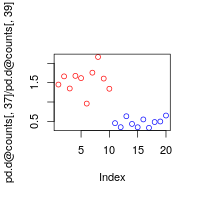This figure shows a clear difference in the ratio abundances between the groups. It also highlights the analogy between disjointed proportionality and differential expression, although the interpretation of differentially abundant ratios differs considerably. Possible biological explanations for this event might include a reduction in the amount of mRNA degradation, a change in isoform splice bias, or an increase in the binding affinity (i.e., $$K_a$$) of a transcriptionally relevant co-factor.

## Emergent proportionality

In contrast, emergent proportionality has more in common with a test for differences in correlation coefficients. That is, emergent proportionality occurs when a pair is proportional in one group but not the other, such that the group with no proportionality contributes most of the total variance.

Below, we use the getResults function again to tabulate important pairwise measurements. Then, we show a scatter plot of the abundance for features “106” and “2”, as colored by the experimental group, with the slopes of the trend lines equal to the ratio means.

tab <- getResults(pd.e)
plot(pd.e@counts[, 106], pd.e@counts[, 2], col = ifelse(pd.d@group == "WA", "red", "blue"))
grp1 <- pd.e@group == "WA"
grp2 <- pd.e@group != "WA"
abline(a = 0, b = pd.e@counts[grp1, 2] / pd.e@counts[grp1, 106], col = "red")
abline(a = 0, b = pd.e@counts[grp2, 2] / pd.e@counts[grp2, 106], col = "blue")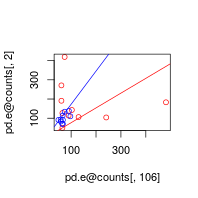Here, we see that these two features change proportionally across the samples within one group but not the other. In other words, the experimental condition appears to have removed the coordination between the transcripts. Moreover, when ignoring the group labels, we see that one group happens to dominate the total VLR. Interestingly, this has happened here without much change in the average abundance ratio, as apparent when viewed through a per-sample projection.

plot(pd.e@counts[, 2] / pd.e@counts[, 106],
col = ifelse(pd.d@group == "WA", "red", "blue"))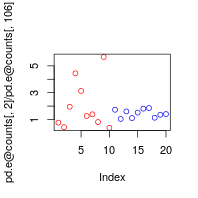This figure confirms that the feature pair varies far more in one group than the other, all while the mean ratio abundances do not change considerably. Note that an increase in $$\theta_e$$ tends to impart a decrease in $$\theta_d$$. Precisely, $$\theta_e$$ relates to $$\theta_d$$ via the function:

$\vartheta_\mathrm{e}=1-\vartheta + \frac{\mathrm{min}[(k-1)\textrm{VLR}_1,(n-k-1)\textrm{VLR}_2]}{(n-1)\textrm{VLR}}$

From this, we establish the inequality:

$1-\vartheta\le\vartheta_\mathrm{e}\le1-\vartheta/2,$

As such, one can use $$1 - \theta_e$$ for a stricter definition of disjointed proportionality. This is implemented in propd as $$\theta_f$$, which one can set active using the setActive function.

pd.f <- setActive(pd, what = "theta_f")
## Alert: Update FDR or F-stat manually.

## Disproportionality networks

A good first step in understanding $$\theta$$ begins with an examination of the disproportionality network. The plot method provides an easy way to build a network such that each circular “node” indicates a transcript while each connecting “edge” indicates an indexed pair (i.e., $$\theta < cutoff$$). Providing a value of $$[0, 1]$$ to the cutoff argument will index pairs based on a maximum value of $$\theta$$. Providing an integer greater than $$1$$ to the cutoff argument will index the top $$N$$ pairs as ranked by $$\theta$$. Note that setting d3 = TRUE will have the rgl package render the network in 3D.

g <- plot(pd.d, cutoff = 1000)
## Red: Pair has higher LRM in group WA than in group QLD
## Blue: Pair has higher LRM in group QLD than in group WA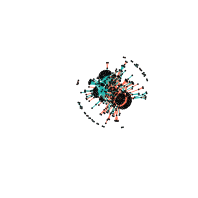For disjointed proportionality networks, red edges show an increase in log-ratio means in Group 1 compared to Group 2 (i.e., increased ratio abundance in Western Australia toads) while blue edges show a decrease in log-ratio means in Group 1 compared to Group 2 (i.e., decreased ratio abundance in Western Australia toads). Importantly, we see that a small number of transcripts participate in a large number of the top differentially proportional pairs.

g <- plot(pd.e, cutoff = 1000)
## Red: Nearly all of total LRV explained by QLD
## Blue: Nearly all of total LRV explained by WA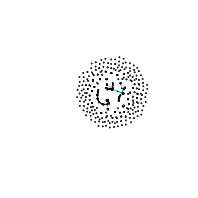For emergent proportionality networks, red edges show an emergence of proportionality in Group 1 compared to Group 2 (i.e., sudden coordination in Western Australia toads) while blue edges show a lack of proportionality in Group 1 compared to Group 2 (i.e., no coordination in Western Australia toads). The architecture of this network is more sparse than the other, making it hard to interpret. However, when viewed in 3D, we can see a few clusters of transcripts that all gained or lost coordination together.

Note that at the appropriate cutoff, a network will contain all pairs for a given FDR. However, we have routinely found that too many pairs remain when using cutoffs with an acceptable FDR. In this case, we recommend stepping through a number of arbitrarily low cutoffs to find one that produces a representative topology that is computationally tractable and human-interpretable.

## Visualizing log-ratio abundance

The parallel function shows the sample-wise distribution of log-ratio abundances for each pair relative to a reference feature. The include argument can specify any feature by name, although users would likely choose a “hub” as the reference. Note that it is not possible to tell from this figure which features have changed in absolute abundance: it is possible for a “hub” to change relative to its neighbors, for all neighbors to change relative to its “hub”, or for a “hub” and its neighbors to have changed simultaneously.

parallel(pd.d, cutoff = .15, include = "c19327_g2_i3")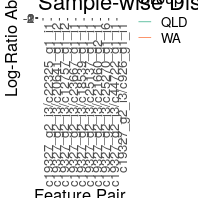parallel(pd.e, include = "c27054_g5_i1")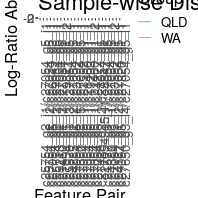## Integrating propd with propr

This package also allows users to integrate the results of proportionality analysis with those from differential proportionality analysis through the union of a propr network and a propd network. We do this with the plot method by passing an indexed propr object to the propr argument. We recommend the companion vignette, “An Introduction to Proportionality” for selecting an appropriate proportionality cutoff. Note that, because CRAN imposes limits on the size of bundled data, as well as the run-time of vignette assembly, we cannot render this figure here. Instead, we provide the code only.

data(caneToad.counts)
keep <- apply(caneToad.counts, 2, function(x) sum(x >= 40) >= 20 & all(x != 0))
plot(pd.e, propr = rho, d3 = TRUE)
Future updates and vignettes will introduce advanced network analyses, precision-weighted $$\theta$$ values, moderated F-statistics, classical hypothesis testing, and more. Stay tuned!